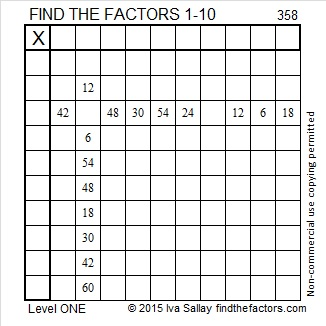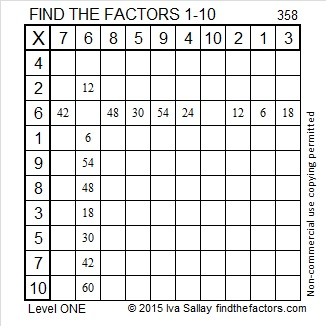# 358 and Level 1

358 is even and therefore a composite number. Scroll down below the puzzle to see its factors.Print the puzzles or type the factors on this excel file: 10 Factors 2015-01-19

• 358 is a composite number.
• Prime factorization: 358 = 2 x 179
• The exponents in the prime factorization are 1 and 1. Adding one to each and multiplying we get (1 + 1)(1 + 1) = 2 x 2 = 4. Therefore 358 has exactly 4 factors.
• Factors of 358: 1, 2, 179, 358
• Factor pairs: 358 = 1 x 358 or 2 x 179
• 358 has no square factors that allow its square root to be simplified. √358 ≈ 18.921This site uses Akismet to reduce spam. Learn how your comment data is processed.